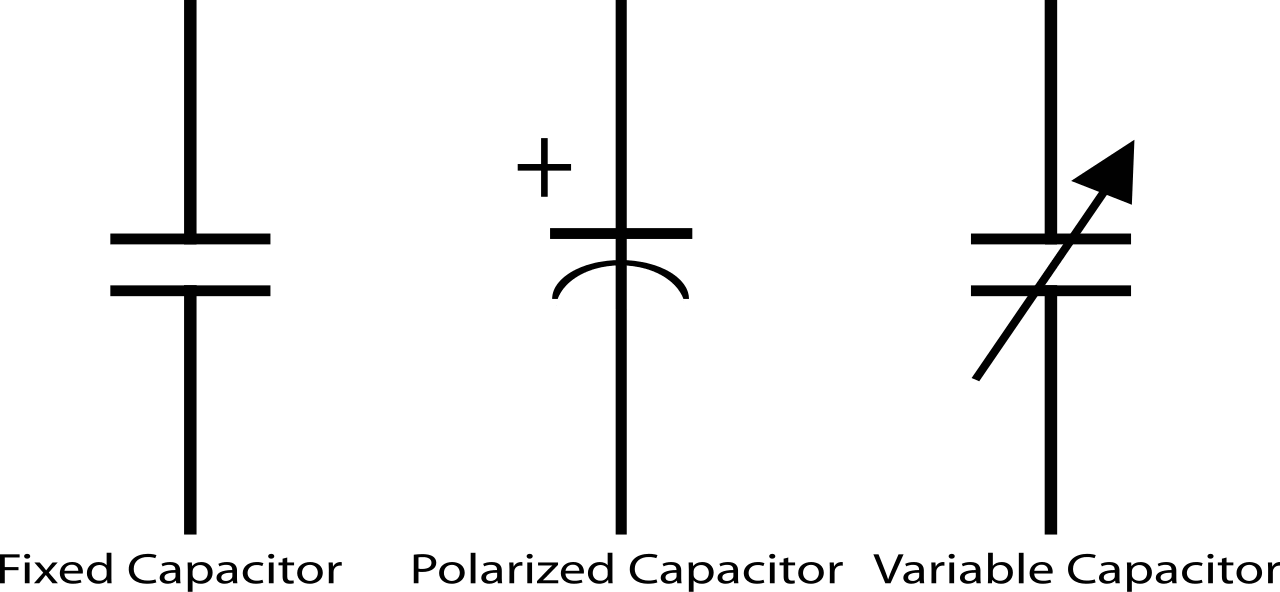This is a Clilstore unit. You can .

# What is electronics?

The electronics is responsible for controlling the movement of electrons carefully and in a controlled manner. It is responsible for passing on more or less passive and active devices.

• Passive devices are: resistors, capacitors and inductors.
• Active devices are diodes, transistors and integrated circuits (semiconductors).

# Resistances

They are used to limit the current passing through various points. The resistance opposing the passage of the current is measured in ohms.

• They are characterized by:

Nominal value: its value in ohms and is marked on the body of the resistor.
Tolerance: plus or minus percentage on the nominal value.
Temperature coefficient: resistance varies with temperature. This variation can be calculated depending on the temperature coefficient.
Power: power that can dissipate the resistor under ambient conditions of 20 to 25C. The greater the more power will be the size of the resistor.
Nominal voltage limit: is the maximum voltage that can withstand, in extreme, resistor.

There are three types of resistors: fixed, variable and dependent.

• Fixed resistors, are characterized by maintaining a fixed ohmic value.The values ​​of these series are standardized and generally how to indicate on the body is through a color code in wire wound resistors the value is written directly.Calculating resistance Color Code

The interpretation of color coding for resistance image (brown, black, red and yellow) is:

1- resistance put in the right way, with tolerance on the right side.

2- we replace each color value.
1st digit = orange = 3
2nd figure = white = 9
Multiplier = red = x100
Tolerance = gold = ± 5%

3- The nominal value will be: Vn = 3900 Ω ± 5%

4º- The maximum and minimum values will be:
- Maximum value = nominal value + setpoint *
Tolerance / 100 = 3900 + 3900 * 5/100 = 4095 Ω
- Minimum value = nominal value - nominal value *
Tolerance / 100 = 3900-3900 * 5/100 = 3705 Ω

Therefore, the actual resistance value will be between 3705 Ω and 4095 Ω.

• Variable resistor or Trimmers, the variation can be rotary or linear.

According to constructively they can be wound, for great powers, or carbon track.
Vary when using a tool called adjustable, whereas when they have a stem to vary them are called potentiometers.•  Dependent resistors, there are four types of dependent resistors:

NTC: negative resistance temperature coefficient. When the temperature of the same ohmic value decreases.
PTC: positive resistance temperature coefficient. As the temperature thereof increases its ohmic value.
LDR: light dependent resistor. When the light intensity increases on the same ohmic value decreases. They are light resistance.
VDR: strain-dependent resistance. When you increase the voltage at its ends ohmic value decreases, and current circulates at their ends. It is used to protect circuits as the excessive current flow it derives this resistance.# Capacitors

The capacitors are formed by two conductive armatures, separated by an electrically conductive (dielectric) that names the wrong type of capacitor material.

They are of various types: ceramic, polyester, electrolytic paper, mica, tantalum, variable and adjustable.

Electrolytic have polarity and must be respected, otherwise the capacitor can explode.The symbols used to represent the capacitors are:Short url:   http://multidict.net/cs/4356